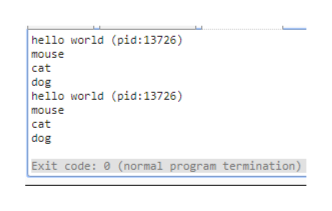# Recall the following code, which we examined in class.v o i d f o r k t e s t ( ){p r i n t f ( ” mouse\n ” ) ;f o r k ( ) ;p r i n t f ( ” c a t\n ” ) ;f o r k ( ) ;p r i n t f ( ” dog\n ” ) ;}i n tmain ( i n t a r g c , c h a r∗a r g v [ ] ){p r i n t f ( ” h e l l o w o r l d ( p i d :%d )\n ” , ( i n t ) g e t p i d ( ) ) ;f o r k t e s t ( ) ;r e t u r n 0 ;}Write down the output of the above program.If you are not able to specify the output of the above program exactly, explain why.

Question
18 views

Recall the following code, which we examined in class.v o i d f o r k t e s t ( ){p r i n t f ( ” mouse\n ” ) ;f o r k ( ) ;p r i n t f ( ” c a t\n ” ) ;f o r k ( ) ;p r i n t f ( ” dog\n ” ) ;}i n tmain ( i n t a r g c , c h a r∗a r g v [ ] ){p r i n t f ( ” h e l l o w o r l d ( p i d :%d )\n ” , ( i n t ) g e t p i d ( ) ) ;f o r k t e s t ( ) ;r e t u r n 0 ;}Write down the output of the above program.If you are not able to specify the output of the above program exactly, explain why.

check_circle

Step 1

The sample output of the ...help_outlineImage Transcriptionclosehello world (pid : 13726) mouse cat dog hello world (pid:13726) mouse cat dog Exit code: 0 (normal program termination) fullscreen

### Want to see the full answer?

See Solution

#### Want to see this answer and more?

Solutions are written by subject experts who are available 24/7. Questions are typically answered within 1 hour.*

See Solution
*Response times may vary by subject and question.
Tagged in

### Operating Systems